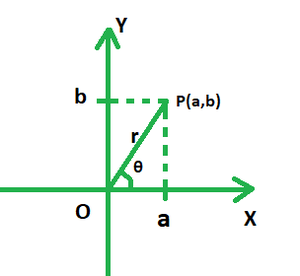# How to use DeMoivre’s Theorem to find the value of i√3?

• Last Updated : 01 Jan, 2022

A complex number can be called a summation of both real and imaginary numbers written or represented usually in the form of z = a + ib, where i (iota) is the imaginary component and represents √(-1). Complex numbers are commonly depicted in their rectangular or standard form as a + ib. For example, 69 + 25i is a complex number where 100 is the real part and 25i is the imaginary part.

Complex numbers can be purely real or purely imaginary depending upon the values of either of each of the two components.

### Polar Form of a Complex Number

To represent a complex number graphically, the polar coordinates of the real and imaginary components are written here. θ represents the angle at which the number line is inclined to the real axis, i.e. the x-axis. The length indicated by the line is known as its modulus, and it is represented by the letter r in the alphabet. The real and imaginary components are represented by a and b, respectively, while the modulus is represented by OP = r in the diagram below.Clearly, we obtain two right triangles in the diagram with perpendiculars along each axis. Applying Pythagoras Theorem would yield the length OP as follows:

r = Modulus[cos(argument) + isin(argument)]

Or, z = r[cosθ + isinθ]

Here,and θ = tan-1(q/p)

### DeMoivre’s Theorem

A polar form is essentially another way of representing a complex integer when its index is 1. DeMoivre’s theorem comes into play when the exponent of a given complex number surpasses one and needs to be evaluated or expanded. To expand a complex number according to its specified exponent, it must first be transformed to its polar form, which has the modulus and argument as constituents. After that, DeMoivre’s theorem is applied, which states:

Formula

For all real values of say, a number x,

(cos x + isinx)n = cos(nx) + isin(nx),

Where n can assume any rational value.

### How to use DeMoivre’s Theorem to find the value of i√3?

Solution:

Modulus = r == 1

Argument = tan-1[1/0] = π/2

Polar Form = r[cosθ + isinθ]Now, i^{√3} =As per DeMoivre’s theorem: (cosθ + isinθ)n = cos(nθ) + isin(nθ).

⇒=.

### Similar Problems

Question 1. Simplify (1 + i)5.

Solution:

Here, r =, θ = π/4

The polar form of (1+i) =According to De Moivre’s Theorem: (cosθ + sinθ)n = cos(nθ) + i sin(nθ).

Thus, (1+i)5= -4 – 4i

Hence, (1 + i)5 = -4 – 4i.

Question 2: Simplify (2 + 2i)6.

Solution:

Here, r =, θ = π/4

The polar form of  (2+2i) =According to De Moivre’s Theorem: (cosθ + sinθ)n = cos(nθ) + isin(nθ).

Thus, (2 + 2i)6= 512 (-i)

Hence, (2 + 2i)6 = −512i.

Question 3: Simplify (1 + i)18.

Solution:

Here, r =, θ = π/4

The polar form of  (1+i) =According to De Moivre’s Theorem: (cosθ + sinθ)n = cos(nθ) + isin(nθ).

Thus, (1+i)18= 512i

Hence, (1 + i)18 = 512i.

Question 4: Simplify (-√3 + 3i)31.

Solution:

Here, r =, θ = 2π/3

The polar form of  (-√3 + 3i) =According to De Moivre’s Theorem: (cosθ + sinθ)n = cos(nθ) + isin(nθ).

Thus, (-√3 + 3i)31Hence, (-√3 + 3i)31 =Question 5. Simplify (1 – i)10.

Solution:

r =, θ = π/4

The polar form of  (1 – i) =According to De Moivre’s Theorem: (cosθ + sinθ)n = cos(nθ) + isin(nθ).

Thus, (1 – i)10= 32 [0 + i(-1)]

= 32 (-i)

Hence, (1 – i)10 = 0 – 32i.

My Personal Notes arrow_drop_up
Recommended Articles
Page :